### Mechanical Aptitude 4 - Levers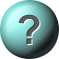1.    In the given picture, if the force arm is shorter?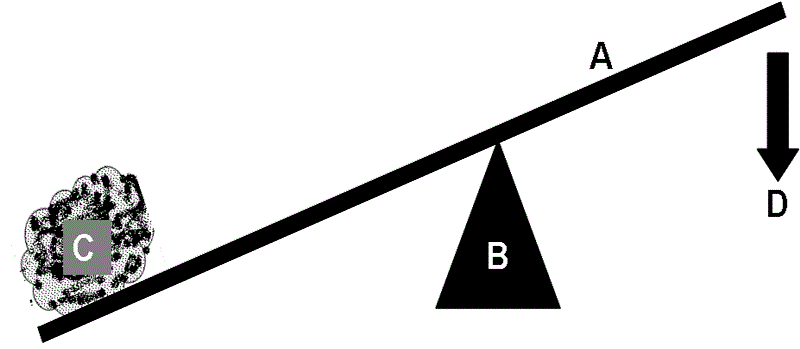A force lesser than the weight will be required to lift the weightA force greater than the weight will be required to lift the weightA force equal to the weight will be required to lift the weightNo answer is correct2.    In the given picture?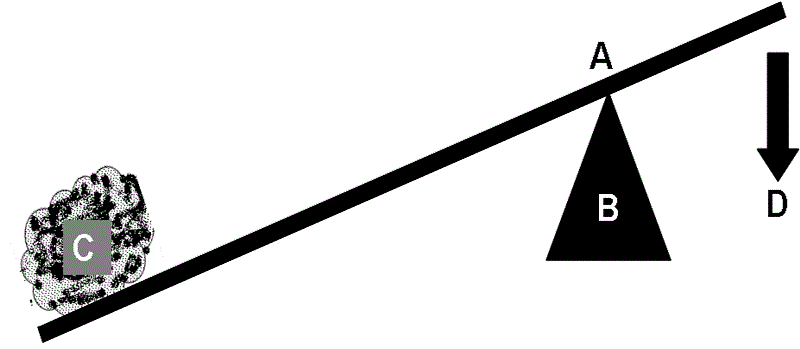A force lesser than the weight will be required to lift the weightA force greater than the weight will be required to lift the weightA force much greater than the weight will be required to lift the weightA force equal to the weight will be required to lift the weight3.    In the given picture?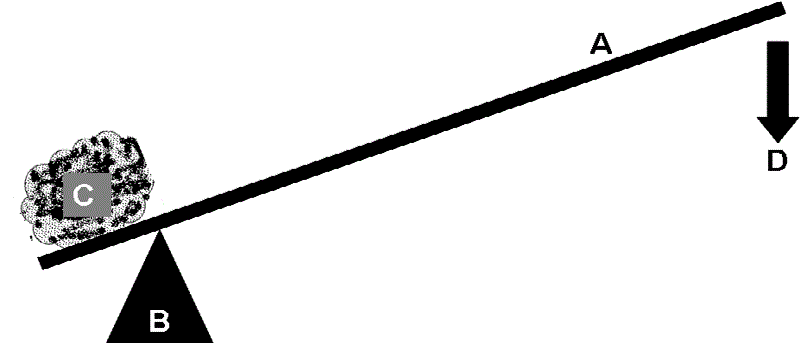A force lesser than the weight will be required to lift the weightA force equal to the weight will be required to lift the weightA force much lesser than the weight will be required to lift the weightA force greater than the weight will be required to lift the weight4.    In the given picture if each weight is 10 KGs?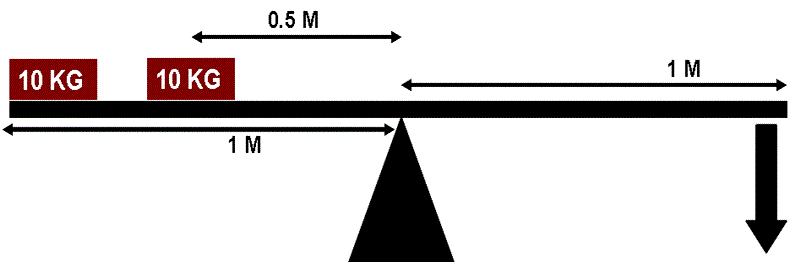A 15 KG force will be required to lift the weightA 5 KG force will be required to lift the weightA 10 KG force will be required to lift the weightA force lesser than 20 KG and more than 10 KG will be required to lift the weight5.    In the given picture if the weight is 20 Kg?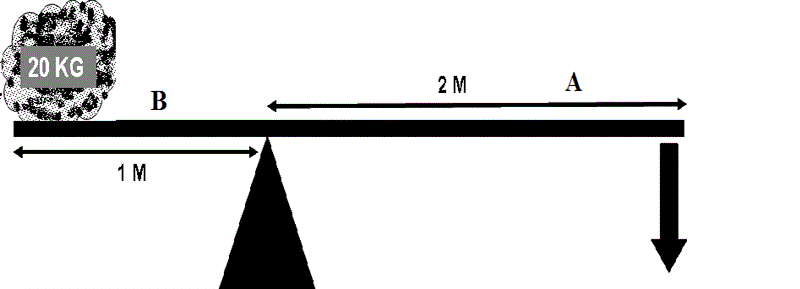A 5 KG force will be required to lift the weightA 10 KG force will be required to lift the weightA 4 KG force will be required to lift the weightA 2.5 KG force will be required to lift the weight

This is more feedback!
This is the feedback!